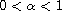Electron. J. Differential Equations, Vol. 2018 (2018), No. 36, pp. 1-10.

### Comparison principles for differential equations involving Caputo fractional derivative with Mittag-Leffler non-singular kernel Mohammed Al-Refai

Abstract:
In this article we study linear and nonlinear differential equations involving the Caputo fractional derivative with Mittag-Leffler non-singular kernel of order. We first obtain a new estimate of the fractional derivative of a function at its extreme points and derive a necessary condition for the existence of a solution to the linear fractional equation. The condition obtained determines the initial condition of the associated fractional initial-value problem. Then we derive comparison principles for the linear fractional equations, and apply these principles for obtaining norm estimates of solutions and to obtain a uniqueness results. We also derive lower and upper bounds of solutions. The applicability of the new results is illustrated through several examples.

Submitted October 14, 2017. Published January 29, 2018.
Math Subject Classifications: 34A08, 35B50, 26A33.
Key Words: Fractional differential equations; maximum principle.

Show me the PDF file (208 KB), TEX file for this article.Mohammed Al-Refai Department of Mathematical Sciences UAE University P.O. Box 15551, Al Ain, UAE email: m_alrefai@uaeu.ac.ae